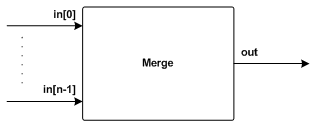CSP for Java
(JCSP) 1.1-rc4

## org.jcsp.plugNplay Class Merge

```java.lang.Objectorg.jcsp.plugNplay.Merge
```
All Implemented Interfaces:
CSProcess

`public final class Mergeextends Objectimplements CSProcess`

Merges an array of strictly increasing Integer input streams into one strictly increasing output stream.

## Process Diagram## Description

Merge is a process whose output stream is the ordered merging of the Integers on its input streams. It assumes that each input stream is strictly increasing (i.e. with no repeats) sequence of Integers. It generates a strictly increasing output stream containing all -- and only -- the Integers from its input streams (eliminating any duplicates).

Warning: this process assumes that its input channel array has at least two elements.

## Channel Protocols

Input Channels
in[] java.lang.Number Assume: in.length >= 2.
All channels can accept data from any subclass of Number. It is possible to send Floats down one channel and Integers down the other. However all values will be converted to ints.
Output Channels
out java.lang.Integer The output will always be of type Integer.

## Example

The following example shows how to use Merge in a small program. The program also uses some of the other plugNplay processes. The program prints, in ascending order (up to Integer.MAX_VALUE), all integers whose prime factors consist only of 2, 3, 5 and 7. Curious readers may like to reason why the infinitely buffered channels are needed.
``` import org.jcsp.lang.*;
import org.jcsp.util.*;
import org.jcsp.plugNplay.*;

public class MergeExample {

public static void main (String[] argv) {

final One2OneChannel[] a = Channel.one2oneArray (5);
final One2OneChannel[] b = Channel.one2oneArray (4, new InfiniteBuffer ());
final One2OneChannel c = Channel.one2one ();
final One2OneChannel d = Channel.one2one ();

new Parallel (
new CSProcess[] {
new Mult (2, a.in (), b.out ()),
new Mult (3, a.in (), b.out ()),
new Mult (5, a.in (), b.out ()),
new Mult (7, a.in (), b.out ()),
new Merge (Channel.getInputArray (b), c.out ()),
new Prefix (1, c.in (), d.out ()),
new Delta (d.in (), Channel.getOutputArray (a)),
new Printer (a.in (), "--> ", "\n")
}
).run ();

}

}
```

## Implementation Note

The implementation sets up a balanced binary tree of parallel Merge2 processes to fan-in the merge from its external input channels to its external output. It's a nice example of recursion and parallelism -- here's the run method:
```   public void run () {
final int n = in.length;  // deduce: n >= 2
switch (n) {
case 2:
new Merge2 (in, in, out).run ();
break;
case 3:
final One2OneChannel c = Channel.one2one();
new Parallel (
new CSProcess[] {
new Merge2 (in, in, c.out ()),
new Merge2 (c.in (), in, out)
}
).run ();
break;
default:  // deduce: n >= 4
final int n2 = n/2;
ChannelInput[] bottom = new ChannelInput[n2];
ChannelInput[] top = new ChannelInput[n - n2];
for (int i = 0; i < n2; i++) {
bottom[i] = in[i];
}
for (int i = n2; i < n; i++) {
top[i - n2] = in[i];
}
final One2OneChannel[] d = Channel.one2oneArray(2);
new Parallel (
new CSProcess[] {
new Merge (bottom, d.out ()),
new Merge (top, d.out ()),
new Merge2 (d.in (), d.in (), out)
}
).run ();
break;
}
}
```

Author:
P.H. Welch
`Merge2`

Constructor Summary
```Merge(ChannelInput[] in, ChannelOutput out)```
Construct a new Merge2 process with the input channels inand the output channel out.

Method Summary
` void` `run()`
The main body of this process.

Methods inherited from class java.lang.Object
`clone, equals, finalize, getClass, hashCode, notify, notifyAll, toString, wait, wait, wait`

Constructor Detail

### Merge

```public Merge(ChannelInput[] in,
ChannelOutput out)```
Construct a new Merge2 process with the input channels inand the output channel out. The ordering of the input channels makes no difference to the behaviour of this process.

Parameters:
`in` - the input channels (there must be at least 2)
`out` - the output channel
Method Detail

### run

`public void run()`
The main body of this process.

Specified by:
`run` in interface `CSProcess`

CSP for Java
(JCSP) 1.1-rc4

Submit a bug or feature to jcsp-team@kent.ac.uk